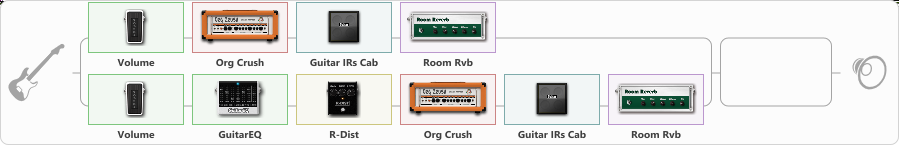# Fikalis

Discussion in 'ToneLib-GFX presets' started by Kek Govnovi, Jun 5, 2022.

1. Fikalis

Preset name: something like black sabbath

single

Effects chain:Effect: "Splitter" (Dynamics / Filter), active - "yes"
{
"A-Bypass" = Off
"A-Pan" = 0
"A-Level" = 55
"B-Bypass" = Off
"B-Pan" = 0
"B-Level" = 55

'A' branch:
{

Effect: "Volume" (Dynamics / Filter), active - "yes"
{
"Pedal Pos." = 34
"Pan" = -47
}

Effect: "Org Crush" (Amp simulators), active - "yes"
{
"Gain" = 28
"Bass" = 60
"Middle" = 50
"Treble" = 71
"Presence" = 77
"Master" = 62
"Level (dB)" = -2
}

Effect: "Guitar IRs Cab" (Cabinets), active - "yes"
{
"Model" = Mesa Rectifier (4x12")
"Mic Position" = Edge
"Mic Distance" = Far
"Low Cut (Hz)" = 0
"Hi Cut (kHz)" = 20.0
"Mix" = 100
"Level (dB)" = 0
}

Effect: "Room Rvb" (Reverb), active - "yes"
{
"Time" = 4.1
"PreDelay" = 16
"LoDamp" = 27
"HiDamp" = 31
"Mix" = 38
}
}
'B' branch:
{

Effect: "Volume" (Dynamics / Filter), active - "yes"
{
"Pedal Pos." = 33
"Pan" = -43
}

Effect: "GuitarEQ" (Dynamics / Filter), active - "yes"
{
"160 Hz" = 0
"400 Hz" = 0
"800 Hz" = 0
"1.6 kHz" = 0
"3.2 kHz" = 2
"6.4 kHz" = 2
"12 kHz" = 3
"Level (dB)" = -2
}

Effect: "R-Dist" (Overdrive / Distortion), active - "yes"
{
"Drive" = 50
"Tone" = 63
"Level" = 49
}

Effect: "Org Crush" (Amp simulators), active - "yes"
{
"Gain" = 34
"Bass" = 60
"Middle" = 50
"Treble" = 71
"Presence" = 78
"Master" = 62
"Level (dB)" = -5
}

Effect: "Guitar IRs Cab" (Cabinets), active - "yes"
{
"Model" = Mesa Rectifier (4x12")
"Mic Position" = Edge
"Mic Distance" = Far
"Low Cut (Hz)" = 44
"Hi Cut (kHz)" = 20.0
"Mix" = 100
"Level (dB)" = 0
}

Effect: "Room Rvb" (Reverb), active - "yes"
{
"Time" = 2.9
"PreDelay" = 16
"LoDamp" = 27
"HiDamp" = 31
"Mix" = 38
}
}
}

Effect: "Splitter" (Dynamics / Filter), active - "yes"
{
"A-Bypass" = Off
"A-Pan" = 0
"A-Level" = 55
"B-Bypass" = Off
"B-Pan" = 0
"B-Level" = 55

'A' branch:
{
}
'B' branch:
{
}
}

Note: You will need to download and install the ToneLib-GFX software to use the preset.

File size:
1.1 KB
Views:
1,826